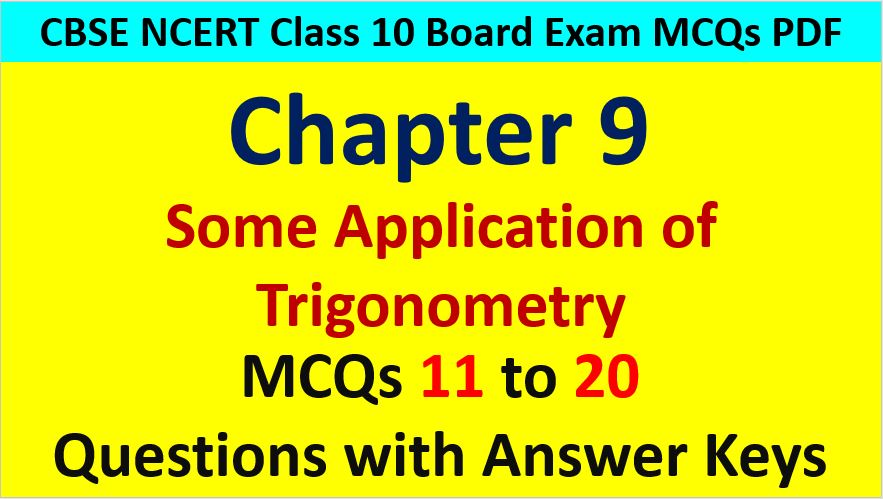Friday, October 22, 2021
Home > CBSE Class 10 > Some Applications of Trigonometry Class 10 Maths MCQ Questions with Answer Keys

# Some Applications of Trigonometry Class 10 Maths MCQ Questions with Answer KeysApplication of Trigonometry MCQ Questions for Class 10 Question No 11:

If the height of a vertical pole is equal to the length of its shadow on the ground, the angle of elevation of the sun is

Option A : 0 degree

Option B : 30 degree

Option C : 45 degree

Option D : 60 degree

Option C : 45 degree

Application of Trigonometry MCQ Questions for Class 10 Question No 12:

If the height of a vertical pole is √3 times the length of its shadow on the ground, then the angle of elevation of the sun at that the time is

Option A : 30 degrees

Option B : 45 degrees

Option C : 60 degrees

Option D : 75 degrees

Option C : 60 degrees

Application of Trigonometry MCQ Questions for Class 10 Question No 13:

If the length of the shadow of a tower is √3 times its height then the angle of elevation of the sun is

Option A : 45 degree

Option B : 30 degree

Option C : 60 degree

Option D : 90 degree

Option B : 30 degree

Application of Trigonometry MCQ Questions for Class 10 Question No 14:

If a pole 12 m high casts a shadow 4√3 m long on the ground then the sun’s elevation is

Option A : 60 degrees

Option B : 45 degrees

Option C : 30 degrees

Option D : 90 degrees

Option A : 60 Degree

Application of Trigonometry MCQ Questions for Class 10 Question No 15:

The shadow of a 5 m long stick is 2 m long. At the same time, the length of the shadow of a 12.5 m high tree is

Option A : 3 m

Option B : 3.5 m

Option C : 4.5 m

Option D : 5 m

Option D : 5 m

Application of Trigonometry MCQ Questions for Class 10 Question No 16:

A ladder makes an angle of 60 degree with the ground when placed a wall. If the foot of the ladder is 2 m away from the wall, then the length of the ladder is

Option A : 4/√3

Option B : 4√3

Option C : 2√2

Option D : 4

Option D : 4

Application of Trigonometry MCQ Questions for Class 10 Question No 17:

A ladder 15 m long makes and angle of 60 degrees with the wall. Find the height of the point, where the ladder touches the wall.

Option A : 15√3 m

Option B : 15√3/2 m

Option C : 15/2 m

Option D : 15 m

Option C : 15/2 m

Application of Trigonometry MCQ Questions for Class 10 Question No 18:

From a point on the ground, 30 m away from the foot of a tower, the angle of elevation of the top of the tower is 30 degree. The height of the tower is

Option A : 30 m

Option B : 10√3 m

Option C : 10 m

Option D : 30√3 m

Option B : 10√3 m

Application of Trigonometry MCQ Questions for Class 10 Question No 19:

The angle of depression of a car parked on the road from the top of a 150 m high tower is 30 degrees. The distance of the car from the tower is

Option A : 50√3 m

Option B : 150√3 m

Option C : 150√2 m

Option D : 75 m

Option A : 50√3 m

Application of Trigonometry MCQ Questions for Class 10 Question No 20:

A kite is flying at a height of 30 m from the ground. The length of string from the kite to the ground is 60 m. Assuming that there is no slack in the string, the angle of elevation of the kite at the ground is

Option A : 45 degrees

Option B : 30 degrees

Option C : 60 degrees

Option D : 90 degrees